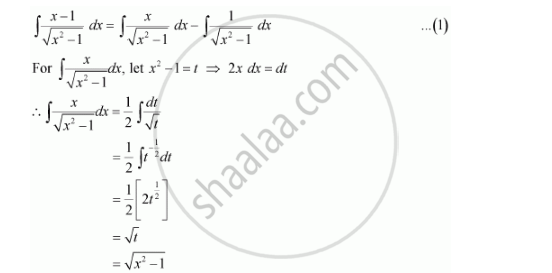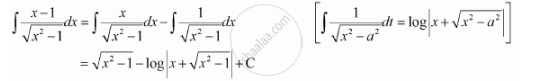Share

# Integrate the Functions (X - 1)/Sqrt(X^2 - 1) - CBSE (Science) Class 12 - Mathematics

ConceptIntegrals of Some Particular Functions

#### Question

Integrate the functions (x - 1)/sqrt(x^2 - 1)

#### SolutionFrom (1), we obtainIs there an error in this question or solution?

#### Video TutorialsVIEW ALL 

Solution Integrate the Functions (X - 1)/Sqrt(X^2 - 1) Concept: Integrals of Some Particular Functions.
S